# 21.## Entering Values and Formulas into a Range

In addition to formatting ranges, you will also create macros that put values and formulas into cells and retrieve values from cells. First you should understand how references work in formulas in Excel, and then you can see how to create formulas in a macro.

### Relative References

Most formulas perform arithmetic operations on values retrieved from other cells. Excel formulas use cell references to retrieve values from cells. Imagine, for example, a list of Retail prices and Wholesale costs.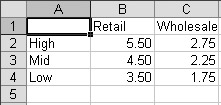Suppose you want to add a column to the list that calculates the gross margin-the difference between the Retail price and the Wholesale cost-for each item. You would put the label Margin in cell D1 and then enter the first formula into cell D2. The formula subtracts the first Wholesale cost (cell C2) from the first Retail price (cell B2). So you would enter =B2-C2 into cell D2.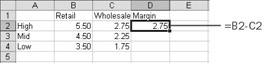For each item in the High group, the gross margin is \$2.75. Now you need to copy the formula to the other rows. The formula you typed into cell D2 refers explicitly to cells C2 and B2. When you copy the formula to cell D3, you want the formula to automatically adjust to refer to C3 and B3. Fortunately, when you copy the formulas, Excel adjusts the references because, by default, references are relative to the cell that contains the formula. (The Prices worksheet in the practice file contains these formulas.)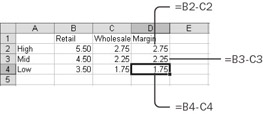If the reference =C2 is found in cell D2, it really means 'one cell to my left.' When you copy the formula to cell D3, it still means 'one cell to my left,' but now that meaning is represented by the reference =C3.

### Absolute References

Sometimes you don't want relative references. Imagine, for example, a worksheet that contains various quantities in column A and prices in row 2.Suppose you want to add formulas to calculate the revenue for each combination. To calculate the first revenue value (cell B3), you need to multiply the first price (cell B2) by the first quantity (cell A3). When you type =B2*A3 into cell B3, you get the correct answer, \$50.

But if you copy that formula to cell B4, you get the ridiculous answer of \$1000. That's because the cell references are relative. In this version of the formula, you're not really referring to cells B2 and A3; you're referring to 'one cell above me' and 'one cell to my left.' When you put the formula into cell B4, 'one cell above me' now refers to cell B3, not cell B2.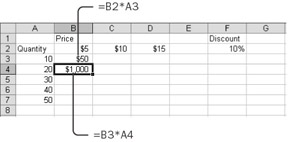You want the prices to adjust from column to column, and you want the quantities to adjust from row to row, but you always want the price to be from row 2 and the quantity to be from column A. The solution is to put a dollar sign (\$) in front of the 2 in the first price reference (B\$2) and in front of the A in the first quantity reference (\$A3). The formula that should go into cell B3 is =B\$2*\$A3. The dollar sign 'anchors' that part of the formula, making it absolute. When you copy the formula to the rest of B3:D7, you get correct answers.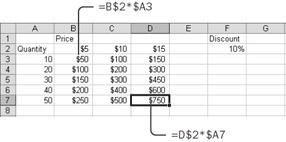The relative portion of the formula changes with the row or column of the cell that contains the formula. The absolute portion remains fixed.

If you want to modify the formula so that it also takes into account the discount value from cell F2, you must make both the row and the column of the discount reference absolute. The correct formula would be =B\$2*\$A3*(1 \$F\$2). (The Revenue worksheet in the practice file contains these formulas.)

### R1C1 Reference Style

As a default, Excel displays letters for column headings and numbers for row headings, and the upper left cell in the worksheet is cell A1. Referring to cells by letter and number is called A1 reference style. In A1 reference style, however, cell references do not really say what they mean. For example, the reference =B3 says 'cell B3,' but it means 'two cells to my left.' You don't know what the reference really means until you know which cell contains the reference.

Excel has an alternate reference style that uses numbers for both column and row headings. In this alternate reference style, to refer to a cell you use the letter R plus the row number and C plus the column number. The upper left cell in the worksheet is therefore R1C1, and referring to cells by row and column numbers is called R1C1 reference style. In R1C1 reference style, cell references really do say what they mean.

To turn on R1C1 reference style, choose the Tools menu, click Options, and then click the General tab. Select the R1C1 Reference Style check box, and click OK. (To turn off R1C1 reference style, clear the check box.) The R1C1 Reference Style check box affects only formulas you enter directly into the worksheet. As you will see in the following section, a macro can enter formulas using either reference style, regardless of the setting in the Options dialog box.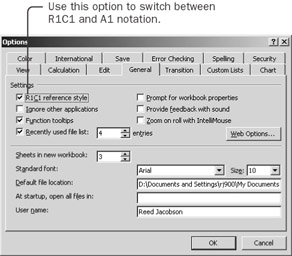In R1C1 reference style, to specify a relative reference on the same row or column as the cell with the formula, you simply use an R or a C, without a number. For example, the reference =RC3 means 'the cell in column 3 of the same row as me,' and the reference =R2C means 'the cell in row 2 of the same column as me.'

To specify a relative reference in a different row or column, you indicate the amount of the difference, in square brackets, after the R or the C. For example, the reference =R5C means 'two columns to my right in row 5,' and the reference =R[-1]C means 'one cell above me.'

The correct formula for calculating the gross margin on the Prices worksheet was =B\$2-\$C2, but only if the formula was entered into cell B2. In R1C1 reference style, the equivalent formula is =R2C-RC1, and it doesn't matter which cell gets the formula. The formula to calculate the discounted price on the Revenue worksheet was =B\$2*\$A3*(1 \$F\$2), at least for cell B2. In R1C1 reference style, the same formula is R2C*RC1*(1 R2C6), again, regardless of the target cell.

 Important When you use A1 reference style, the formula changes depending on which range you copy the formula into. When you use R1C1 reference style, the formula is the same, regardless of which cell it goes into.

Put Values and Formulas into a Range

You can explore the properties for putting values and formulas into a range by creating a simple list of incrementing numbers.

1. In the Visual Basic Editor, activate the Immediate window, type Worksheets.Add and press Enter to create a new, blank worksheet in the active workbook.

2. Type Range("B2:B6").Select and press Enter to select a sample starting range of cells.3. Type Selection.Value = 100 and press Enter to fill all the cells of the selection with the number 100.

Value is a property of the range. When you set the Value property in conjunction with a multicell range, you change all the cells in the range.

 Tip You could just as well have used Selection.Formula = 100 to assign the constant to the cell. The Formula property is equivalent to whatever you see in the formula bar when the cell is selected. The formula bar can contain constants as well as formulas, and so can the Formula property. When you assign a value to a cell, the Formula property and the Value property have the same effect.
4. Type ActiveCell.Value = 0 and press Enter to change cell B2 to zero.

Only the active cell changes, not the selected cells. Entering a value in the active cell is equivalent to typing a value and pressing Enter. Entering a value in the selection is equivalent to typing a value and pressing Ctrl+Enter.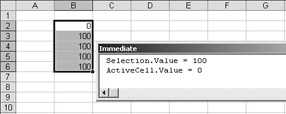Suppose you want to enter a value in the cell above the active cell, whatever the active cell might be.

5. Type ActiveCell.Offset(-1).Value = 1 and press Enter statement to change cell B1 to 1.

This statement starts with the active cell, uses the Offset property to calculate a new cell one up from that starting cell, and then sets the Value property for the resulting cell.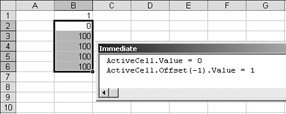6. Type Selection.FormulaR1C1 = "=R[-1]C*5" and press Enter.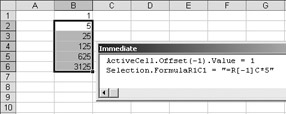Now each of the selected cells contains a formula, not a constant. The FormulaR1C1 property expects a formula in R1C1 reference style. The reference R[-1]C always means 'one cell above' regardless of which cell is active when you enter the formula.

7. Type ?ActiveCell.Value and press Enter.

This statement displays the value 5 in the Immediate window. The Value property retrieves the result of any formula in a cell. When you retrieve the contents of the cell that contains a formula, the Value property gives you the result of the formula.

8. Type ?ActiveCell.Formula and press Enter.

This statement displays the formula =B1*5 in the Immediate window. When you retrieve the contents of a cell that contains a formula, the Formula property gives you the formula using A1 reference style. The setting in the Excel Options dialog box is ignored. If you want to retrieve the formula using R1C1 reference style, use the FormulaR1C1 property.

All cells have Formula, FormulaR1C1, and Value properties. The Value property and the Formula property are the same when you're writing to the cell. When you read the value of a cell, the Value property gives you the value, and the Formula property gives you the formula using A1 reference style. The FormulaR1C1 property is the same as the Formula property, except that it uses all references in R1C1 reference style, whether assigning a formula to the cell or reading the formula from a cell.

 Tip The Value property always gives you the unformatted value of the number in a cell. A cell also has a Text property, which returns the formatted value of the cell. The Text property is read- only because it's a combination of the Value property and the NumberFormat property.

Use the Address of a Range to Build Formulas

Sometimes you need a macro to create formulas that contain references. For example, suppose you have a range of cells such as the one on the Data worksheet and you want totals at the bottom of the two values columns. If the size of the range can change, you don't know until the macro runs which cells should be included in the SUM formula.

Sometimes the list, with headings, might extend from row 2 to row 4, in which case the formula for the values in the Units column should be =SUM(G3:G4) in cell G5. Another time, the range of cells might extend from row 2 to row 10, in which case the formula should be =SUM(G3:G10), this time in cell G11. Interactively, you would use the AutoSum button to create the formulas, but using the AutoSum button while recording a macro always generates formulas with specific addresses. You can't use the AutoSum button if you need to create a macro that can handle ranges of differing sizes. You need a macro that behaves like a simplified version of the AutoSum button.

1. In Excel, select the Data worksheet. Then activate the Visual Basic Editor, choose the Insert menu, click Module, and enter the following macro framework:

`Sub MakeTotals()     Dim myData As Range     Dim myTotal As Range     Set myData = Range("B2").CurrentRegion     Set myData =          myData.Offset(1, 5).Resize(myData.Rows.Count-1, 2)     Set myTotal = myData.Rows(myData.Rows.Count + 1)     End Sub`
2. Press F8 repeatedly until the End Sub statement is the next statement. In the Immediate window, type myData.Select and press Enter. Then type myTotal.Select and press Enter. The variables should contain references to the ranges G3:H14 and G15:H15, respectively. The expressions used to refer to the range all come from the 'Referring to a Range' section earlier in this chapter. Your goal is to put a formula into each cell of the myTotal range that sums all the values from the corresponding column of the myData range.3. Activate the Object Browser, and select the Range class. In the list of Members, select Address. The Address property returns a string and takes five arguments, each of which is optional.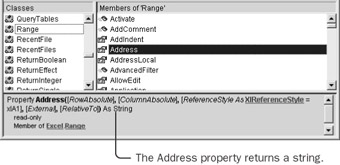4. In the Immediate window, type ?myData.Columns(1).Address and press Enter.

This statement displays the reference \$G\$3:\$G\$14. This is the address of the first column of the data range. If you were to put this address inside a SUM function, the total would be correct for the first total, but the dollar signs mean that the reference is absolute. You need to make the column reference relative so that it will adjust as you put it into both columns of the totals row. The first two arguments of the Address property control whether the row and column references are absolute. The default value for each argument-True-produces an absolute reference.

5. Type ?myData.Columns(1).Address(True,False) and press Enter.

This statement displays the reference G\$3:G\$14. This statement tells the Address property to make row references absolute but column references relative. You can now put this reference into the SUM function, creating a formula that is suitable for adding to the total range.

6. Type ?"=SUM(" & myData.Columns(1).Address(True,False) & ")" and press Enter.

This statement displays the formula =SUM(G\$3:G\$14). This is the final formula you will assign to the total range. The expression joins the first part of the SUM function with the reference returned by the Address property, and then adds the closing parenthesis. An ampersand character (&) joins pieces of text together.

7. Choose the View menu, and click the Last Position command.

This takes you to the macro where the End Sub statement is still the next statement.

8. Before the End Sub statement, insert the statement myTotal.Formula = "=SUM(" & myData.Columns(1).Address(True, False) & ")". Then drag the Next Statement arrow back up to the new statement, and press F8 to execute it.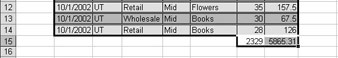The totals appear in the row at the bottom, appropriately different for each column.

9. Press F8 to finish the macro, and then press F5 to run it again, adding a second row of totals.

This is a little silly, but it shows how the macro automatically adjusts as new rows are added to the data. The CurrentRegion property includes any new rows. The Address property calculates the appropriate reference for the SUM function.

Ranges are a powerful tool in Excel. You can select ranges, assign them to variables, add formulas to them, name them, and retrieve their addresses. By manipulating ranges, you can build powerful, dynamic worksheet models.

 Tip When you construct a formula by using the Address property of a range, the easiest approach is usually to use A1 reference style and assign the formula to the Formula property of the target range. When you enter a finished formula as a string into a macro statement, the safest approach is usually to use R1C1 reference style and assign the formula to the FormulaR1C1 property of the target range. (This is what the macro recorder does.) To convert a formula from A1 to R1C1 reference style, record a macro as you enter the A1 reference style formula into a cell.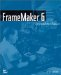FrameMaker 6: Beyond the Basics
ISBN: 735613591
EAN: 2147483647
Year: 2005
Pages: 122
Authors: Lisa Jahred

Similar book on Amazon